# Substance-field Models for Fourth Class StandardsRelated Tools & Articles
 Discussion Forum
 "Altshuller steadily tried to improve TRIZ with new tools and methods and each time he thought the last one was the best. To me, it’s a rather a sign of enthusiasm than of rejection..."Contribute to this Discussion

## Abstract:

Theory of Inventive Problem Solving (TRIZ) standards are used for finding inventive solutions to everyday problems. These standards include a set of generalized rules and formulas for solving common problems that are found in work environments. They also contain clear instructions for how an initial technical system should be transformed in order to solve the problem. The problem solving standards are a powerful tool in TRIZ for individuals who understand each standard. It is difficult, however, for new people to grasp the concept because each standard is just a sentence with a short comment with no corresponding graphics.

The substance-field theory was conceptualized by the founding father of TRIZ, Genrich Altshuller. He did not provide graphic models for every standard; however, graphic models help illustrate the main idea of each standard, especially for beginners. Graphic models for standards in this class would be helpful. This paper presents a method for individuals to see all graphic models for fourth class standards.

## Keywords

TRIZ, standards, graphic model

## 1. Introduction

The problem solving tool of TRIZ is a system made up of 76 standard solutions. These standard solutions include generalized rules and formulas for solving specific work environment problems. The standards contain clear instructions for how an initial technical system should be transformed in order to solve the problem. Therefore, inventive solutions are used to solve typical problems. The generalized rules (or standard solutions) provide recommendations based on the analysis of past inventions that solved similar problems.

Substance-field models can be considered the minimal language of TRIZ and can be represented by substance-field models in a graphic way. During the training process these graphic models offer a helpful visual tool for TRIZ beginners to see the substance-field models as they correspond to the 76 standard solutions. This is especially useful when trying to understand fourth class solutions since Altshuller did not provide substance-field models for them. In Altshuller's book, Creativity as the Exact Science, he shows 18 typical substance-field models and transformations.1 (It is not known why Altshuller did not provide visual graphs for all substance-field solutions for fourth class.)

## 2. Fourth Class Differences

There are several essential differences among the fourth class and the first three classes of standards. A breakdown of the first three classes:

1. Building and destruction of substance-fields: dedicated to solving the problems necessary to construct or transform substance-field models
2. Development of substance-fields: related to solving problems through the evolution of substance-field models
3. Transition to super-system and micro-level: is used for problem solving with the development of a solution on the super-system or subsystem level2

The goal of the first three classes is to increase the effectiveness of the current system by adding or changing something. The basic graphic model of the three classes, therefore, consists of three elements – two substances and a field – to form a triangle.

The goal of this model (triangle) is to express the function of a system by describing the interaction among these three elements as seen in Figure 1.

 Figure 1: Basic Substance-field Model of the First Three Classes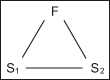The fourth class of standards, "standards for detection and measuring," is dedicated to solving measuring problems or problems that have the specific purpose of detecting something.

If the goal of the fourth class is the same as the first three classes, it would improve the effectiveness of measuring a technical system. In some ways that would be right. The essential goal of the fourth class, however, is to obtain information from the object to be measured or detected – gather the correct information. For example, take the value of a parameter of the measured/detected object from the object that should be measured/detected. The graphic model of the fourth class should express the method of "how to get the object's information."

After seeing the essential differences among the fourth class and the first three classes, is the triangle in Figure 1 suitable for expressing the core idea of the fourth class?

## 3. What Is information and How Does Information Flow During the Measurement Process?

Norbert Wiener, the founder of cybernetics said: "Information is information not matter or energy." This statement suggests that information should be considered along with matter and energy as the third constituent part of the universe, where information is carried by matter or energy. Here, substance can be seen as matter and field can be seen as energy.

The value of the parameter needed from the measured/detected object is objective. The object that is measured/detected is a carrier of the value (objective information). The goal is to perform a measurement/detection to get the value. But individuals can not get the exact value just like they can never get an exact truth. Individuals must get the value by measuring/detecting an indirect phenomenon. This phenomenon may latch on to some kind of field or substance. but it includes information about the value needed. (See Figure 2)

 Figure 2: Information Transfer Process When Measuring the Length of a Cuboid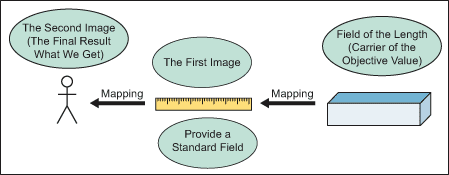Figure 2 shows a simple measuring example. The objective value of the length of the cuboid is the result when using a ruler to measure the length of the cuboid. The measurement process can be expressed in the following way:

1. The ruler can be regarded as a sensor and it provides a standard field of length. At the same time, the length of the cuboid is another field. This field is a carrier of the objective value of the length. Compare the field of the length of the cuboid with the standard field of the ruler to measure the length of the cuboid. The result is a ratio of these two fields. The name of this ratio can be called "first time image of the objective value of the length."
2. Take a reading of this ratio from the ruler and visualize the image. The name of this image can be called "second time image of the objective value of the length." Usually the "second time image of the objective value of the length" is the resulting value of the length of the cuboid.

The measurement action can be expressed by accurately mapping it two times. The measurement process for the information flow is detailed in Figure 3:

 Figure 3: Information Flow in the Measurement Process## 4. Setting Up Substance-field Models

In Figure 3 the model includes all elements connected with measurement action. Not all of them, however, are a prerequisite for the set up of the substance-field model for the fourth class. For example, standard solutions for the fourth class do not consider the person who performs the measurement action. The image of the ratio that is produced in a person's mind is not considered either. Therefore, these two elements (S3 and F3) can be omitted as shown in Figure 4.

 Figure 4: Omitting Elements "Person" and "Image of the Ratio"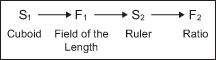In this model (Figure 4) S1 is a substance (or object) which contains the information (called target information) that individuals want to know. Usually, target information is an objective value or state of a parameter of the object.

F1 is a field that is generated by S1. A substance can generate many fields. The fields that contain information (first derivative information of the target information) can be a candidate for F1 – F1 is a carrier of first derivative information.

S2 is a sensor. It must provide a standard field that is able to sense and measure the derivative information in F1. Its primary function is to achieve a ratio between derivative information and a standard field. This ratio is considered second derivative information of the target information. Consequently, S2 is a producer of second derivative information. When S2 produces a ratio it must transfer or express this ratio into a simple form in order to sense and understand. Finally, S2 exports this "simple form" information (called third derivative information) with some kind of field.

F2 is the carrier of third derivative information and is generated by S2. It acts directly on human senses and must be easy to understand.

 Figure 5: Information CarriersFigure 5 shows four elements. Since S2 is considered a measurement tool or sensor, it is not important in TRIZ. There are special disciplines in engineering colleges that research how to design sensors and how to generate an easy field. S2 and F2 can be deleted (or omitted) from the model in Figure 5. But some changes of the S2 sensor need to happen regarding the standard solutions in 4.1.3 and 4.5.1, respectively. When only F2 is deleted (or omitted) the result, shown in Figure 6, is:

 Figure 6: The Most Essential Model of the Fourth ClassIn Figure 6 the model is simple and illustrates the essential core model of the fourth class. The final goal of most standard solutions for the fourth class is to solve the problem of "how to make S1 produce a good field, which contains the first derivative information with the highest quality."

Figure 7 shows the problem model of most standard solutions of the fourth class.

 Figure 7: Problem Model of Most Standard Solutions of Fourth Class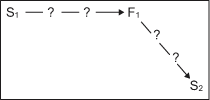In Figure 7 the arrows with the "?" denote that S1 produces F1, but F1 does not contain enough information (first derivative information) for the sensor to measure/detect. F1 is, therefore, considered a weak field.

In most situations the object that was measured/detected can not generate a "good" field by itself. The object must be excited with a field (F0) from an outside system or from the sensor. The basic problem model for the default field is shown in Figure 8.

 Figure 8: Basic Problem Model with a Default Field## 5. Main Symbols of Graphic Models for Fourth Class

The following symbols are used to build substance-field graphic models that correspond to standard solutions for the fourth class:

 Table 1: Main Symbols of Graphic Models for the Fourth Class Symbol Fourth Class Meaning S Substance: an object, device, assembly, component, material of the component or its element. S1 is the object to be measured/detected. S2 is a sensor. F Field: an acting force or a field. The concept of a field includes mechanical (including gravitational and acoustic), chemical, electric, magnetic and nuclear types of interactions with interacting substances. It also represents energy or information. F1 is the field that was generated by S1. F1 contains the first derivative information.Desired action or effect: between S1 and F1 this symbol means S1 generates F1; at the same time, F1 is a high quality field, which contains enough information (first derivative information) of the target information.Lack of informationInsufficient (or inefficient) desired action or effect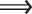Solution operator

## 6. Graphic Substance-field Models of the Fourth Class

Now, all substance-field models corresponding to the 17 standard solutions of the fourth class are available.

## 4.1. Indirect Methods

### 4.1.1 Replacing Detection or Measurement with System Change

If there is a problem with detection or measurement then it is appropriate to modify the system in a way that renders the need for solving the problem obsolete.

 Figure 9: Replacing Detection or Measurement with System Change (4.1.1)### 4.1.2 Applying Copies

If there is a problem with detection or measurement and it is impossible to apply the standard solution in 4.1.1 then it is appropriate to manipulate a copy or photo of an object instead of the object itself.

 Figure 10: Applying Copies (4.1.2)As shown in Figure 10: F0 is different from F0', and F1 is different from F1', and S2 is different from S2', because S1 is different from S1copy.

### 4.1.3 Measurement as Two Consecutive Detections

If there is a problem with detection or measurement and it is impossible to apply the standard solutions of 4.1.1 and 4.1.2, respectively then it is appropriate to transform the problem into one where two consecutive detections of change are carried out.

Where F1' may or may not be the same as F1''

 Figure 11: Measurement as Two Consecutive Detections (4.1.3)## 4.2. Building Measurement Substance-field Models

### 4.2.1 Measurement of a Substance-field Model

If an incomplete substance-field model is difficult to measure or detect then the problem can be solved by completing a regular or double substance-field model with a field output, as shown in Figure 12, follows:

 Figure 12: Measurement of a Substance-Field Model (4.2.1)Figure 13: Standard Solution Model (4.2.1.1)4### 4.2.2. Complex Measurement of a Substance-field Model

This can be used if a system or its parts is difficult to detect, or if measuring the problem can be solved by transitioning to the internal or external complex substance-field model using easily-detected additives. Consider Figure 14, where Sa is the additive.

 Figure 14: Complex Measurement of a Substance-Field Model (4.2.2)Or consider Figure 15, where F is the field between S1 and Sa.

 Figure 15: Standard Solution Model (4.2.2.1)2### 4.2.3. Measurement Substance-field Model with the Environment

If a system is difficult to detect or measure at certain moments in time and it is impossible to introduce additives, then additives capable of generating an easily-detected (or easily-measured) field should be introduced into the environment. Changes in the state of the environment will provide information about changes in the system as follows: where Sa is the additive, where Senv is the environment and where Fenv is the field that is generated by the environment. (Figure 16)

 Figure 16: Measurement Substance-field Model with the Environment (4.2.3)Figure 17: Standard Solution Model (4.2.3.1)2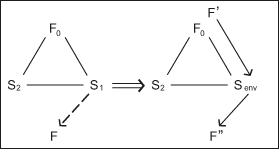### 4.2.4 Obtaining Additives in the Environment

If it is impossible to introduce additives into the environment in accordance with standard solution 4.2.3 then these additives can be produced in the environment by itself; this can be accomplished through its destruction or by changing its phase state. In particular, gas or vapor bubbles obtained by electrolysis, cavitation or other methods are often applied. For example, Figure 18 shows Sda derived by additives in the environment.

 Figure 18: Measurement Substance-field Model with the Environment (4.2.4)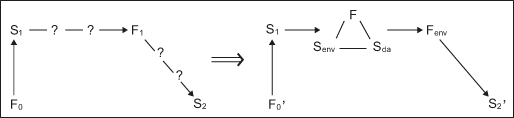## 4.3 Enforcing Measurement Substance-field Models

### 4.3.1 Applying Physical Effects and a Phenomenon

The effectiveness of a measurement and/or detection in a substance-field model can be enhanced by utilizing a physical phenomenon. For example, Figure 19 represents a situation where effect is the physical phenomenon.

 Figure 19: Applying Physical Phenomenon (4.3.1)### 4.3.2 Applying Resonance Oscillations of a Sample

If it is impossible to directly detect or measure changes in a system and passing a field through the system is impossible, then the problem can be solved by generating resonance oscillations. Either as a part of the system or as a whole, variations in oscillation frequency provide information about changes in the system. Figure 20 displays the situation where Fresonance is a field generated by resonance oscillations.

 Figure 20: Applying Resonance Oscillations of a Sample (4.3.2)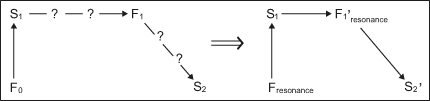Figure 21: Standard Solution Model (4.3.2.1)2### 4.3.3. Applying Resonance Oscillations of a Joined Object

If applying standard solution 4.3.2 is impossible, then information about the state of the system can be obtained via free oscillations of an external object or by the environment linked to the system.

 Figure 22: Applying Resonance Oscillations of a Joined Object (4.3.3)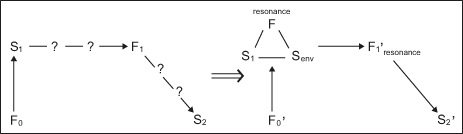Because the joined object resides in the environment, Senv is used to express the environment, which also includes the joined object.

## 4.4. Transition to Ferro-field Models

### 4.4.1. Measurement of a Pre-Ferro-field Model

Substance-field models with non-magnetic fields are apt to be transformed into pre-Ferro-field models containing magnetic substances and a magnetic field.

Where S1'mag is S1 with a magnetic property;
Where F1'mag is a magnetic field, which is generated by S1'mag:

 Figure 23: Measurement of a Pre-Ferro-field Model (4.4.1)### 4.4.2 Measurement of a Ferro-field Model

The effectiveness of a measurement and/or detection of a substance-field model or pre-Ferro-field model can be enhanced by transitioning to a Ferro-field model. This can be done by substituting one of the substances with ferromagnetic particles or by adding ferromagnetic particles. Information is then obtainable via detection or measurement of the magnetic field, as shown in Figure 24:

 Figure 24: : Measurement of a Ferro-Field Model (4.4.2)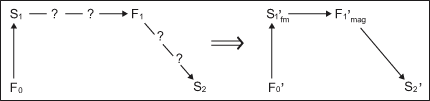In this standard solution S1 is a mixed substance, in other words, S1 = Smain + Sother. This standard solution substitutes Sother with ferromagnetic particles or adds ferromagnetic particles into the mixed substance.

Consider this example:

S1 = Smain + Sother » S1' = Smain + Sfm

Or this example:

S1 = Smain + Sother » S1' = Smain + Sother + Sfm

However, compare this standard solution with Figure 26 and the standard solution is to substitute Sother with ferromagnetic particles.
In Figure 24 the S1'fm means to substitute one of the substances (Sother) with ferromagnetic particles (Sfm) or add ferromagnetic particles (Sfm) into S1.
For example, as shown in Figure 25:

S1 = S + Sother

And the Sother is substituted by ferromagnetic particles, which have magnetic properties (Smfm).

 Figure 25: Standard Solution Model (4.4.2.1)2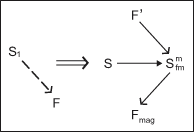### 4.4.3 Complex Measurement of a Ferro-field Model

If the effectiveness of a measurement and/or detection can be enhanced by transitioning to a Ferro-field model, but a substance substitution with Ferromagnetic particles is prohibited, then this transition can be performed by creating a complex Ferro-field model through the introduction of additives into the substance as shown in Figure 26:

 Figure 26: Complex Measurement Ferro-field Model (4.4.3)Figure 27: Standard Solution Model (4.4.3.1)2### 4.4.4 Measurement of a Ferro-field Model with the Environment

If the effectiveness of a measurement and/or detection can be enhanced by transitioning to a Ferro-field model, but the introduction of ferromagnetic particles is prohibited, then the particles should be introduced into the environment.

For example, where Fenvmag is a magnetic field, which is generated by the environment:

 Figure 28: Measurement of a Ferro-field Model with the Environment (4.4.4)### 4.4.5 Applying Physical Effects and a Phenomenon

The effectiveness of a measurement and/or detection substance-field or a pre-Ferro-field model can be enhanced by applying a physical phenomenon such as the Curie effect (a curie is a unit of radiological measurement), the Hopkinson law (which states that the magneto motive force is equal to the product of the magnetic flux and the reluctance of the circuit), and the Barkhausen effect (which suggests ferromagnetic materials contain regions of like-oriented atoms), magnetoelasticity, etc.

 Figure 29: Applying Physical Effects and a Phenomenon (4.4.5)## 4.5 Evolution Direction of Measurement Systems

### 4.5.1 Transition to Bi- or Poly-systems

The effectiveness of a measurement and/or detection substance-field or pre-Ferro-field model at any stage of evolution can be enhanced by building a bi- or poly-system.

 Figure 30: Transition to Bi- or Poly-Systems (4.5.1)### 4.5.2 Evolution Direction

Systems for measurement and/or detection evolve in three directions:

1. Measuring a function,
2. Measuring the first derivative of the function or
3. Measuring the second derivative of the function.

 Figure 31: Evolution Direction (4.5.2)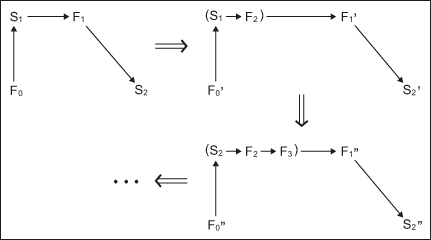## Conclusion

The substance-field model is the basis of a substance-field analysis and standard solution system. It is a useful tool for identifying problems in a technical system and for finding solutions to problems. The methods in this article describe the setup of all standard substance-field model solutions for the fourth class. This method is not perfect, but it is a beginning. These models will help TRIZ beginners easily understand and visualize standard solutions.

## References

1. Nataliya N. Narbut, Alexander Th. Narbut. "TRIZ: History of the Instruments," TRIZ Center, February 2008.
2. Kraev, Val, "Kraev's Korner: Inventive Standards & S-field Models - Lesson 8," The TRIZ Journal, May 2007.
3. Philatov, V., Zlotin, B., Zusman, A., Altshuller, G., (1999). Tools of Classical TRIZ, Ideation International Inc., March 1999.
4. Official G.S. Altshuller Foundation's website, TRIZ Standards.